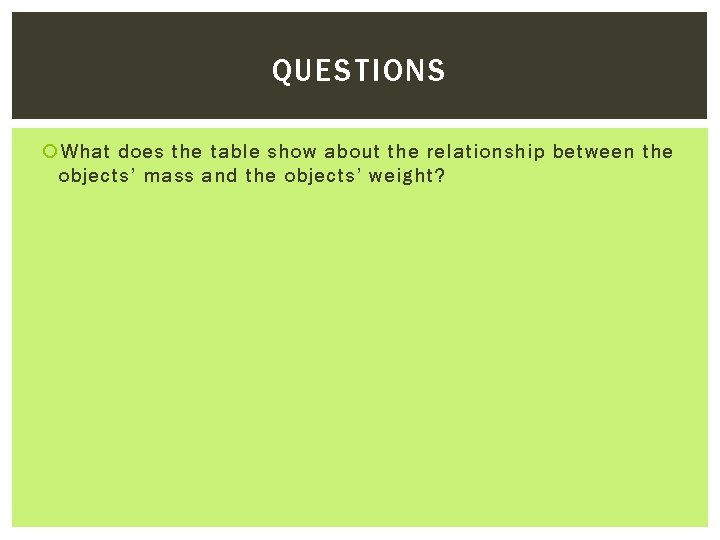MASS AND WEIGHT MASS AND WEIGHT Mass is

• Slides: 21MASS AND WEIGHT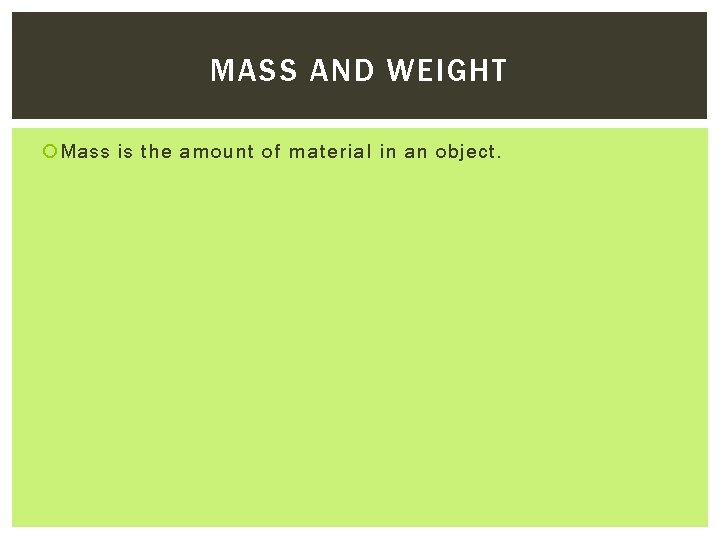MASS AND WEIGHT Mass is the amount of material in an object.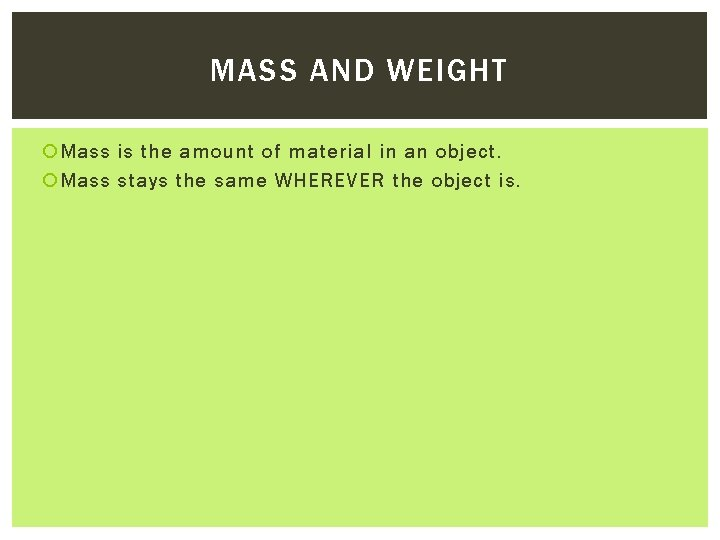MASS AND WEIGHT Mass is the amount of material in an object. Mass stays the same WHEREVER the object is.MASS AND WEIGHT Mass is the amount of material in an object. Mass stays the same WHEREVER the object is. Mass is measured in kilograms (kg) or grams (g)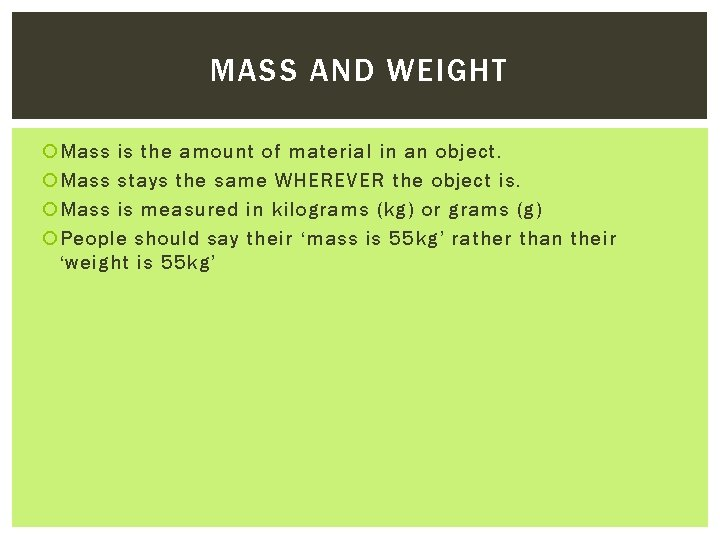MASS AND WEIGHT Mass is the amount of material in an object. Mass stays the same WHEREVER the object is. Mass is measured in kilograms (kg) or grams (g) People should say their ‘mass is 55 kg’ rather than their ‘weight is 55 kg’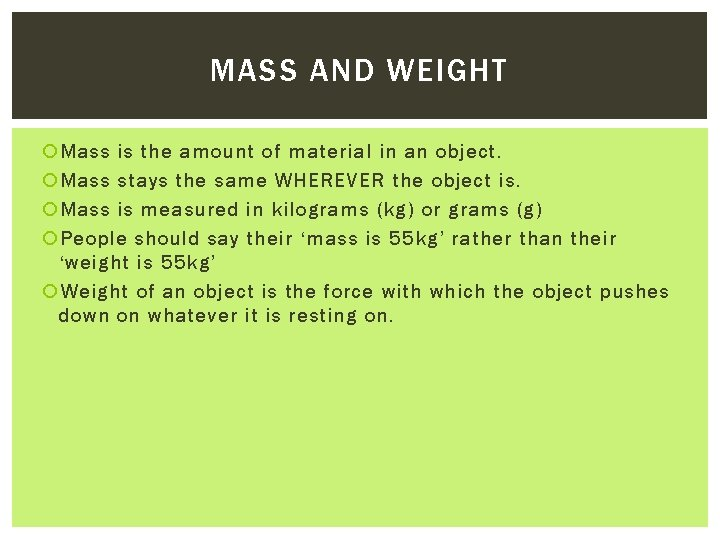MASS AND WEIGHT Mass is the amount of material in an object. Mass stays the same WHEREVER the object is. Mass is measured in kilograms (kg) or grams (g) People should say their ‘mass is 55 kg’ rather than their ‘weight is 55 kg’ Weight of an object is the force with which the object pushes down on whatever it is resting on.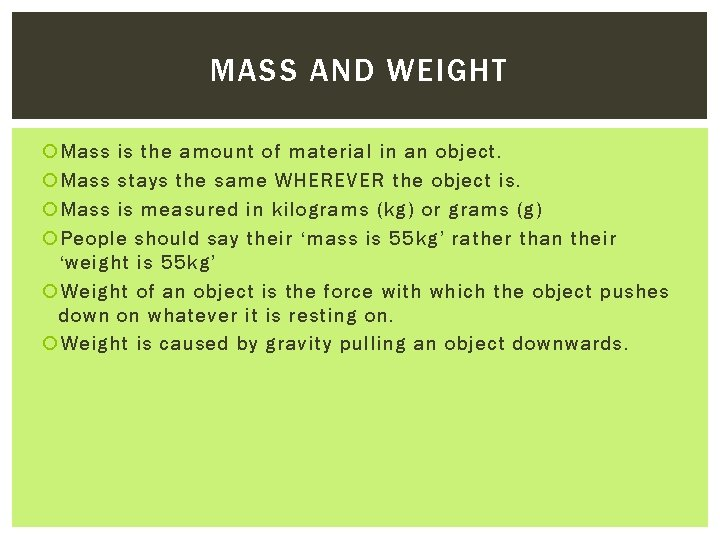MASS AND WEIGHT Mass is the amount of material in an object. Mass stays the same WHEREVER the object is. Mass is measured in kilograms (kg) or grams (g) People should say their ‘mass is 55 kg’ rather than their ‘weight is 55 kg’ Weight of an object is the force with which the object pushes down on whatever it is resting on. Weight is caused by gravity pulling an object downwards.MASS AND WEIGHT Mass is the amount of material in an object. Mass stays the same WHEREVER the object is. Mass is measured in kilograms (kg) or grams (g) People should say their ‘mass is 55 kg’ rather than their ‘weight is 55 kg’ Weight of an object is the force with which the object pushes down on whatever it is resting on. Weight is caused by gravity pulling an object downwards.MASS AND WEIGHT Mass is the amount of material in an object. Mass stays the same WHEREVER the object is. Mass is measured in kilograms (kg) or grams (g) People should say their ‘mass is 55 kg’ rather than their ‘weight is 55 kg’ Weight of an object is the force with which the object pushes down on whatever it is resting on. Weight is caused by gravity pulling an object downwards. The gravity force of an object is often called the weight force because they both have the same size.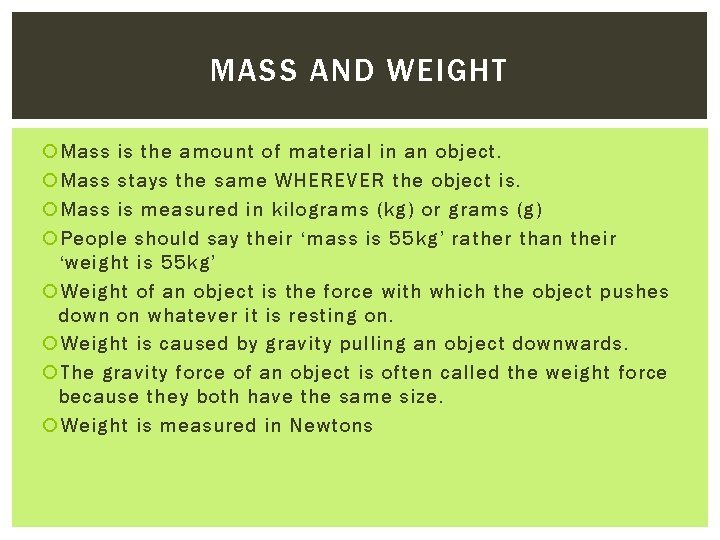MASS AND WEIGHT Mass is the amount of material in an object. Mass stays the same WHEREVER the object is. Mass is measured in kilograms (kg) or grams (g) People should say their ‘mass is 55 kg’ rather than their ‘weight is 55 kg’ Weight of an object is the force with which the object pushes down on whatever it is resting on. Weight is caused by gravity pulling an object downwards. The gravity force of an object is often called the weight force because they both have the same size. Weight is measured in Newtons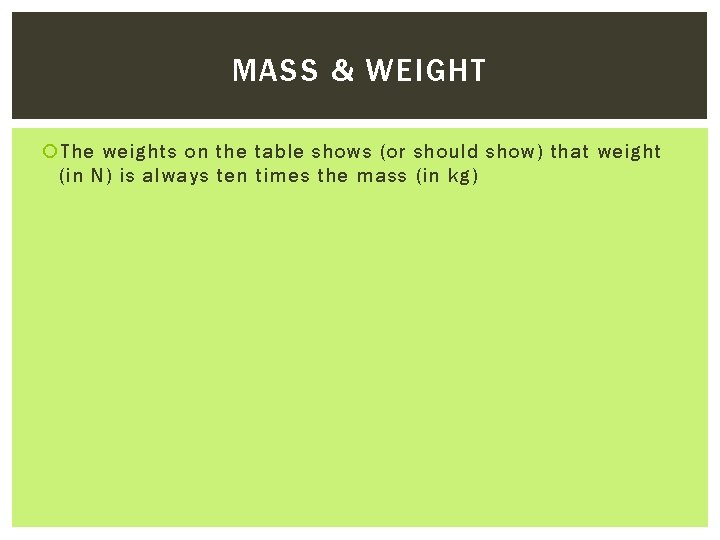MASS & WEIGHT The weights on the table shows (or should show) that weight (in N) is always ten times the mass (in kg)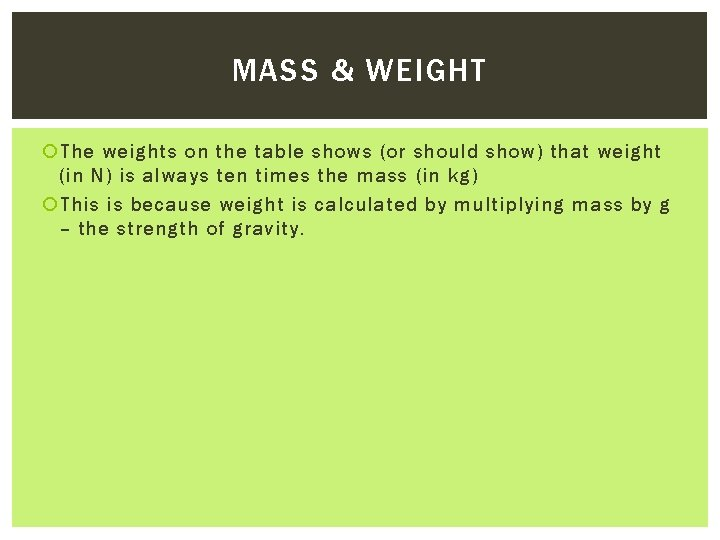MASS & WEIGHT The weights on the table shows (or should show) that weight (in N) is always ten times the mass (in kg) This is because weight is calculated by multiplying mass by g – the strength of gravity.MASS & WEIGHT The weights on the table shows (or should show) that weight (in N) is always ten times the mass (in kg) This is because weight is calculated by multiplying mass by g – the strength of gravity. The value of g (gravity) Is 10 Newtons per kilogram (10 kg -1) on the surface of the earth.MASS & WEIGHT The weights on the table shows (or should show) that weight (in N) is always ten times the mass (in kg) This is because weight is calculated by multiplying mass by g – the strength of gravity. The value of g (gravity) Is 10 Newtons per kilogram (10 kg -1) on the surface of the earth. This means that every kg of mass pushes down with a weight of 10 N.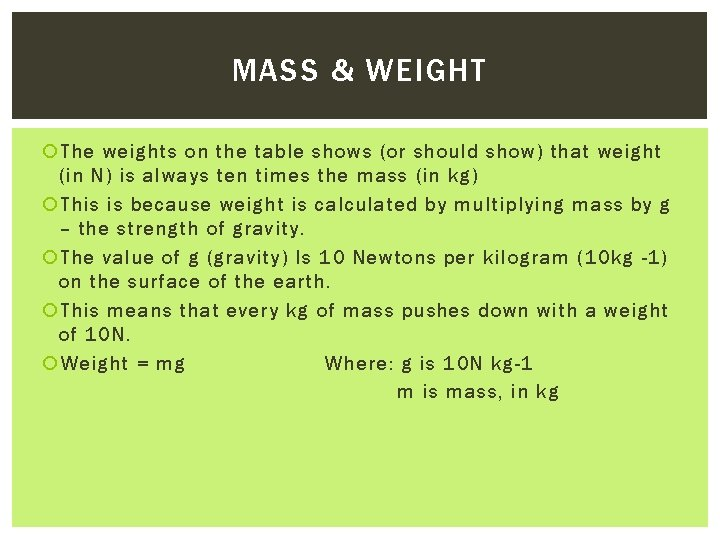MASS & WEIGHT The weights on the table shows (or should show) that weight (in N) is always ten times the mass (in kg) This is because weight is calculated by multiplying mass by g – the strength of gravity. The value of g (gravity) Is 10 Newtons per kilogram (10 kg -1) on the surface of the earth. This means that every kg of mass pushes down with a weight of 10 N. Weight = mg Where: g is 10 N kg-1 m is mass, in kgMASS & WEIGHT The weights on the table shows (or should show) that weight (in N) is always ten times the mass (in kg) This is because weight is calculated by multiplying mass by g – the strength of gravity. The value of g (gravity) Is 10 Newtons per kilogram (10 kg -1) on the surface of the earth. This means that every kg of mass pushes down with a weight of 10 N. Weight = mg Where: g is 10 N kg-1 m is mass, in kg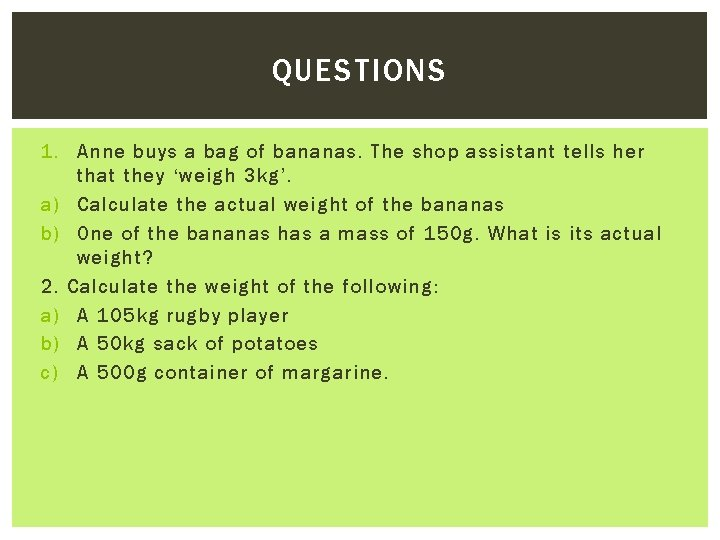QUESTIONS 1. Anne buys a bag of bananas. The shop assistant tells her that they ‘weigh 3 kg’. a) Calculate the actual weight of the bananas b) One of the bananas has a mass of 150 g. What is its actual weight? 2. Calculate the weight of the following: a) A 105 kg rugby player b) A 50 kg sack of potatoes c) A 500 g container of margarine.QUESTIONS 3. A 15 kg rock is dislodged from a cliff and falls to the ground below. a) Calculate the force of gravity pulling the rock downwards b) What is the force of the rock on the ground at the foot of the cliff after it has landed?COMPLETE THE TABLE FROM THE PRACTICAL In pairs, get a force meter and use the weights to find out mass and weight. Remember!!! You must convert the mass into kg… Mass (kg) Weight (N)